# 2. 盒子滤波¶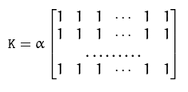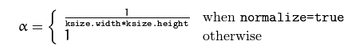# 3. 原始实现¶

void BoxFilterOrigin(float *Src, float *Dest, int Width, int Height, int Radius){
for(int Y = 0; Y < Height; Y++){
for(int X = 0; X < Width; X++){
int ST_Y = Y - Radius;
if(ST_Y < 0) ST_Y = 0;
int EN_Y = Y + Radius;
if(EN_Y > Height-1) EN_Y = Height-1;
int ST_X = X - Radius;
if(ST_X < 0) ST_X = 0;
int EN_X = X + Radius;
if(EN_X > Width-1) EN_X = Width-1;
float sum = 0;
for(int ty = ST_Y; ty <= EN_Y; ty++){
for(int tx = ST_X; tx <= EN_X; tx++){
sum += Src[ty * Width + tx];
}
}
Dest[Y * Width + X] = sum;
}
}
}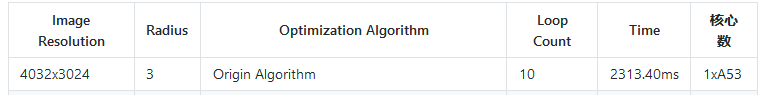# 2. 第一版优化¶

void BoxFilterOpenCV(float *Src, float *Dest, int Width, int Height, int Radius, vector <float>&cache){
float *cachePtr = &(cache);
// chuizhi
for(int Y = 0; Y < Height; Y++){
for(int X = 0; X < Width; X++){
int ST_X = X - Radius;
if(ST_X < 0) ST_X = 0;
int EN_X = X + Radius;
if(EN_X > Width-1) EN_X = Width-1;
float sum = 0;
for(int tx = ST_X; tx <= EN_X; tx++){
sum += Src[Y * Width + tx];
}
cachePtr[Y * Width + X] = sum;
}
}

//shuiping
for(int Y = 0; Y < Height; Y++){
int ST_Y = Y - Radius;
if(ST_Y < 0) ST_Y = 0;
int EN_Y = Y + Radius;
if(EN_Y > Height-1) EN_Y = Height-1;
for(int X = 0; X < Width; X++){
float sum = 0;
for(int ty = ST_Y; ty <= EN_Y; ty++){
sum += cachePtr[ty * Width + X];
}
Dest[Y * Width + X] = sum;
}
}
}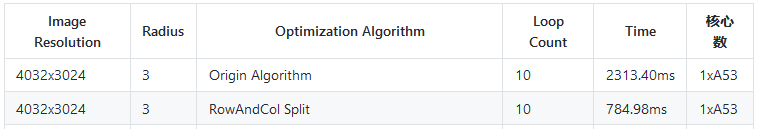# 3. 第二版 优化¶

void BoxFilterOpenCV2(float *Src, float *Dest, int Width, int Height, int Radius, vector<float>&cache){
float *cachePtr = &(cache);
//chuizhi
for(int Y = 0; Y < Height; Y++){
int Stride = Y * Width;
float sum = 0;
for(int X = 0; X < Radius; X++){
sum += Src[Stride + X];
}
for(int X = 0; X <= Radius; X++){
sum += Src[Stride + X + Radius];
cachePtr[Stride + X] = sum;
}
//middle
sum += Src[Stride + X + Radius];
sum -= Src[Stride + X - Radius - 1];
cachePtr[Stride + X] = sum;
}
//tail
for(int X = Width - Radius; X < Width; X++){
sum -= Src[Stride + X - Radius - 1];
cachePtr[Stride + X] = sum;
}
}
//shuipin

for(int X = 0; X < Width; X++){
float sum = 0;
for(int Y = 0; Y < Radius; Y++){
sum += cachePtr[Y * Width + X];
}
for(int Y = 0; Y <= Radius; Y++){
sum += cachePtr[Y * Width + Radius * Width + X];
Dest[Y * Width + X] = sum;
}
//middle
sum += cachePtr[Y * Width + Radius * Width + X];
sum -= cachePtr[Y * Width - (Radius + 1) * Width + X];
Dest[Y * Width + X] = sum;
}
//tail
for(int Y = Height-Radius; Y < Height; Y++){
sum -= cachePtr[Y * Width - (Radius + 1) * Width + X];
Dest[Y * Width + X] = sum;
}
}
}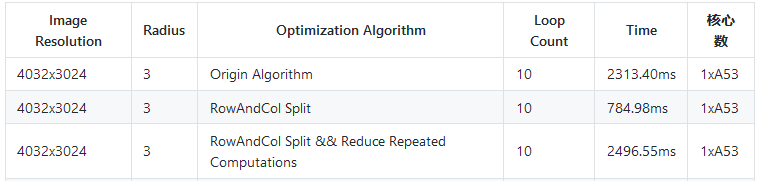# 4. 第三版优化 减少Cache Miss¶

void BoxFilterCache(float *Src, float *Dest, int Width, int Height, int Radius, vector<float>&cache){
float *cachePtr = &(cache);
//chuizhi
for(int Y = 0; Y < Height; Y++){
int Stride = Y * Width;
float sum = 0;
for(int X = 0; X < Radius; X++){
sum += Src[Stride + X];
}
for(int X = 0; X <= Radius; X++){
sum += Src[Stride + X + Radius];
cachePtr[Stride + X] = sum;
}
//middle
sum += Src[Stride + X + Radius];
sum -= Src[Stride + X - Radius - 1];
cachePtr[Stride + X] = sum;
}
//tail
for(int X = Width - Radius; X < Width; X++){
sum -= Src[Stride + X - Radius - 1];
cachePtr[Stride + X] = sum;
}
}

vector <float> colsum;
colsum.resize(Width);
float *colsumPtr = &(colsum);
for(int X = 0;  X < Width; X++){
colsumPtr[X] = 0;
}
//shuipin
for(int Y = 0; Y < Radius; Y++){
int Stride = Y * Width;
for(int X = 0; X < Width; X++){
colsumPtr[X] += colsumPtr[Stride + X];
}
}
for(int Y = 0; Y <= Radius; Y++){
int Stride = Y * Width;
for(int X = 0; X < Width; X++){
colsumPtr[X] += cachePtr[(Y + Radius) * Width + X];
Dest[Stride + X] = colsumPtr[X];
}
}
//middle
int Stride = Y * Width;
for(int X = 0; X < Width; X++){
colsumPtr[X] += cachePtr[(Y + Radius) * Width + X];
colsumPtr[X] -= cachePtr[(Y - Radius - 1) * Width + X];
Dest[Stride + X] = colsumPtr[X];
}
}
//tail
for(int Y = Height-Radius; Y < Height; Y++){
int Stride = Y * Width;
for(int X = 0; X < Width; X++){
colsumPtr[X] -= cachePtr[(Y - Radius - 1) * Width + X];
Dest[Stride + X] = colsumPtr[X];
}
}
}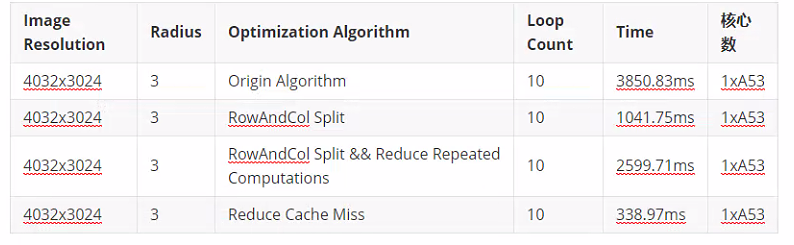# 5. 第四版优化 Neon Intrinsics¶

    int Block = Width >> 2;
int Remain = Width - (Block << 2);

//Origin
// for(int Y = 0; Y < Radius; Y++){
//     int Stride = Y * Width;
//     for(int X = 0; X < Width; X++){
//         colsumPtr[X] += colsumPtr[Stride + X];
//     }
// }
for(int Y = 0; Y < Radius; Y++){
int Stride = Y * Width;
float* tmpColSumPtr = colsumPtr;
float* tmpCachePtr = cachePtr;

int n = Block;
int re = Remain;

for(; n > 0; n--){
float32x4_t colsum = vld1q_f32(tmpColSumPtr);
float32x4_t cache = vld1q_f32(tmpCachePtr);

vst1q_f32(tmpColSumPtr, sum);

tmpColSumPtr += 4;
tmpCachePtr += 4;
}

for (; re > 0; re--) {
*tmpColSumPtr += *tmpCachePtr;
tmpColSumPtr ++;
tmpCachePtr ++;
}
}


NEON 内置函数命名方式有两种，分别对应源操作数是否涉及标量，具体解释如下。
1）源操作数涉及标量时，数据类型表示为v op dt_n/lane_type。

①n表示源操作数是标量而返回向量，lane 表示运算涉及向量的一个元素。
③dt是目标向量和源向量长度表示符。

④type表示源数据类型缩写，如u8 表示 uint8；u16 表示 uint16；u32 表示 uint32；s8 表示 int8；s16 表示 int16；s32 表示 int32；f32 表示 float32。
2）源操作数全是向量时，数据类型表示为v op dt_type，其中op、dt和type的含义和源操作数为标量时一致。

1）内置函数vmla_f32表示使用64位向量寄存器操作32位浮点数据，即源操作数使用的向量寄存器和目标操作数使用的向量寄存器表示都是float32x2_t。
2）内置函数vmlaq_f32表示使用128位向量寄存器操作32位浮点数据，即源操作数使用的向量寄存器和目标操作数使用的向量表示都是float32x4_t。
3）内置函数vmlal_u32表示使用的目标寄存器是128位向量，源寄存器是64位向量，操作32位无符号整数。
5）内置函数vmovn_u64表示目标寄存器是64位向量，源寄存器是128位向量，即同时操作两个数。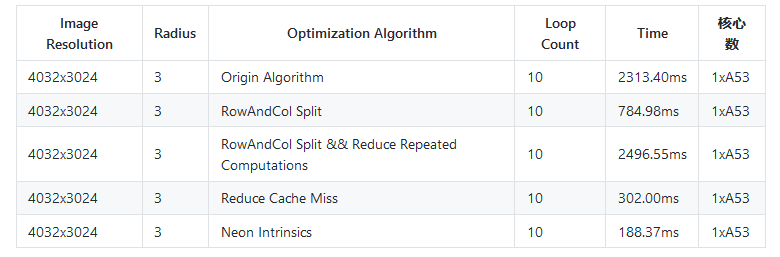可以看到使用Neon Intrinsics之后，速度又有了进一步提升，接下来的一步需要向Neon Assembly以及调度板子上拥有的2块A53角度去分析了。

# 6. 内联汇编入门¶

## 6.1 armv7/v8 寄存器介绍¶

ARM是微处理器行业的一家知名企业，其芯片结构有：armv5、armv6、armv7和armv8系列。芯片类型有：arm7、arm9、arm11、cortex系列。指令集有：armv5、armv6和neon指令。具体可以参考：http://baike.baidu.com/view/11200.htm

### 通用寄存器¶

armv7有16个32-bit的通用寄存器，用R0-R15来表示，而armv8有31个64-bit的通用寄存器，用X0-X30 来表示，还有一个不同名字的特殊寄存器，用途取决于上下文,，因此我们可以看成 31个64位的X寄存器或者31个32位的W寄存器(X寄存器的低32位)。如下图所示：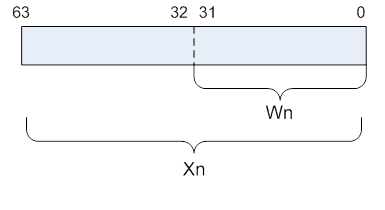### 向量寄存器¶

armv7包含16个128-bit的向量寄存器，用Q0-Q15来表示，其中每个寄存器又可以当作两个64-bit的向量寄存器来使用，用D0-D31来表示，对应关系为：

$Q_n=(D_{n*2},D_{n*2+1})$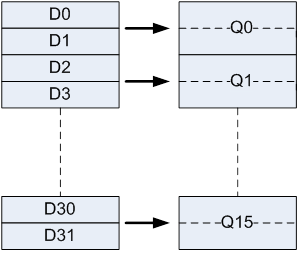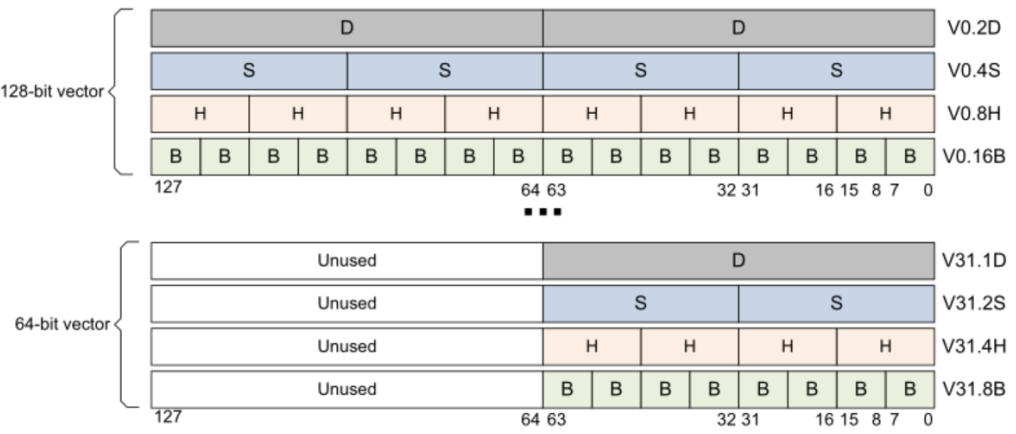从上图的上半部分来看，每一个128-bit的V寄存器都可以当做

• 2个64-bit的向量寄存器，用Vn.2D来表示，注意n代表寄存器的下标，图中展示了0和31两种情况。
• 4个32-bit的向量寄存器，用Vn.4S来表示。
• 8个16-bit的向量寄存器，用Vn.8H来表示。
• 16个8-bit的向量寄存器，用Vn.16B来表示。

• 1个64-bit的向量寄存器，用Vn.1D来表示。
• 2个32-bit的向量寄存器，用Vn.2S来表示。
• 4个16-bit的向量寄存器，用Vn.4H来表示。
• 8个8-bit的向量寄存器，用Vn.8B来表示。

## 6.2 内联汇编一般格式¶

asm asm-qualifiers ( AssemblerTemplate
: OutputOperands
: InputOperands
: Clobbers)


• AssemblerTemplate：代表我们需要自己实现的汇编代码部分。

• OutputOperands： 代表在内联汇编中会被修改的变量列表，变量之间用逗号隔开。然后每个变量的格式表示为：[asmSymbolicName] "constraint"(cvariablename) ,其中：

• cvariablename：表示变量原始的名字。
• asmSymbolicName：表示变量在内联汇编代码中的别名，一般和cvariablename一样，在汇编代码部分就可以通过%[asmSymbolicName]去使用这个变量。
• constraint：这个比较复杂可以填的参数很多，例如填r就表示如果寄存器操作数在通用寄存器中，则允许使用该操作数。更详细可以看https://gcc.gnu.org/onlinedocs/gcc/Extended-Asm.html#OutputOperands
• InputOperands : 代表在内联汇编中用到的所有变量列表（包含会被修改和无须修改的），变量之间仍用逗号隔开。每个变量的格式为：[asmSymbolicName] "constraint"(cexpression)。和上面介绍的OutputOperands 不一样的地方在于，首先需要按照OutputOperands列表的顺序将变量再列一遍，但是constraint用数字代替从0开始，然后才是写其他只读变量，只读变量constraintr

• Clobbers：一般以"cc", "memory"开头，然后接着填内联汇编中用到的通用寄存器和向量寄存器。其中cc表示内联汇编代码修改了标志寄存器，而memory则通知GCC当前内联汇编语句可能会对某些寄存器或内存进行修改，希望GCC在编译时能够将这一点考虑进去。

#if __ARM_NEON
int nn = size >> 2;
int remain = size - (nn << 2);
#else
int remain = size;
#endif // __ARM_NEON

#if __ARM_NEON
#if __aarch64__
if (nn > 0)
{
asm volatile(
"0:                               \n"
"prfm       pldl1keep, [%1, #128] \n"
"ld1        {v0.4s}, [%1]         \n"
"fabs       v0.4s, v0.4s          \n"
"subs       %w0, %w0, #1          \n"
"st1        {v0.4s}, [%1], #16    \n"
"bne        0b                    \n"
: "=r"(nn), // %0
"=r"(ptr) // %1
: "0"(nn),
"1"(ptr)
: "cc", "memory", "v0");
}
#else
if (nn > 0)
{
asm volatile(
//汇编代码部分
"0:                             \n"
"vld1.f32   {d0-d1}, [%1]       \n"
"vabs.f32   q0, q0              \n"
"subs       %0, #1              \n"
"vst1.f32   {d0-d1}, [%1]!      \n"
"bne        0b                  \n"
//OutputOperands
: "=r"(nn), // %0
"=r"(ptr) // %1
//InputOperands
: "0"(nn),
"1"(ptr)
//Clobbers 这里只有q0这个向量寄存器
: "cc", "memory", "q0");
}
#endif // __aarch64__
#endif // __ARM_NEON
for (; remain > 0; remain--)
{
*ptr = *ptr > 0 ? *ptr : -*ptr;

ptr++;
}
}


# 7. 第五版优化 Neon内联汇编¶

int n = Block;
int re = Remain;

// for(; n > 0; n--){
//     float32x4_t colsum = vld1q_f32(tmpColSumPtr);
//     float32x4_t sub = vld1q_f32(tmpsubPtr);
//     sum = vsubq_f32(sum, sub);

//     vst1q_f32(tmpColSumPtr, sum);
//     vst1q_f32(tmpDestPtr, sum);

//     tmpsubPtr += 4;
//     tmpColSumPtr += 4;
//     tmpDestPtr += 4;
// }
// 我的翻译顺序为OutputOperands->InputOperands->汇编代码->Clobbers

asm volatile(
"0:                       \n" //开头0标记，类似do while中的while(n>0)里的0
//浮点数每个32位，乘以四就是128位。最后感叹号表示，这个指令完成之后

"vld1.s32 {d2-d3}, [%1]!  \n" //同理，处理tmpsubPtr，放到q1寄存器
"vld1.s32 {d4-d5}, [%2]   \n" //同理，处理tmpColSumPtr，放到q2寄存器，由于tmpColSumPtr要改变值
//，所以暂时不移动地址，等待计算完成再移动

"vsub.f32 q3, q4, q1      \n" //对应sum = vsubq_f32(sum, sub);

"vst1.s32 {d6-d7}, [%3]!  \n" //把寄存器的内容存到tmpDestPtr地址指向的内存
"vst1.s32 {d6-d7}, [%2]!  \n" //把寄存器的内容存到tmpColSumPtr地址指向的内存
"subs %4, #1              \n" //n-=1
"bne  0b                  \n" //bne判断nn是否为0， 不为0则继续循环跳到开头0标记出继续执行
// OutputOperands
"=r"(tmpsubPtr),
"=r"(tmpColSumPtr),
"=r"(tmpDestPtr),
"=r"(n)
// InputOperands
"1"(tmpsubPtr),
"2"(tmpColSumPtr),
"3"(tmpDestPtr),
"4"(n)
//Clobbers 这里用到了q0,q1,q2,q3,q4这五个向量寄存器
: "cc", "memory", "q0", "q1", "q2", "q3", "q4"
);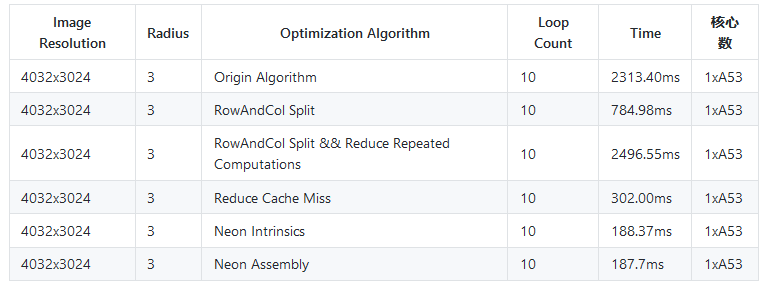# 8. 第六版优化 ARM中的预取命令pld的使用¶

pld，即预读取指令，pld指令只在armv5以上版本有效。使用pld指令可以提示ARM预先把cache line填充好。pld指令中的offset很有讲究。一般为64-byte的倍数。 功能：cache预读取（PLD,PreLoad），使用pld指示存储系统从后面几条指令所指定的存储器地址读取，存储系统可使用这种方法加速以后的存储器访问。 格式pld[Rn,{offset}] 其中： Rn 存储器的基址寄存器。 Offset 加在Rn上的偏移量。

// 我的翻译顺序为OutputOperands->InputOperands->汇编代码->Clobbers

asm volatile(
"0:                       \n" //开头0标记，类似do while中的while(n>0)里的0
"pld      [%0, #128]      \n"
//浮点数每个32位，乘以四就是128位。最后感叹号表示，这个指令完成之后
"pld      [%1, #128]      \n"
"vld1.s32 {d2-d3}, [%1]!  \n" //同理，处理tmpsubPtr，放到q1寄存器
"pld      [%2, #128]      \n"
"vld1.s32 {d4-d5}, [%2]   \n" //同理，处理tmpColSumPtr，放到q2寄存器，由于tmpColSumPtr要改变值
//，所以暂时不移动地址，等待计算完成再移动

"vsub.f32 q3, q4, q1      \n" //对应sum = vsubq_f32(sum, sub);

"vst1.s32 {d6-d7}, [%3]!  \n" //把寄存器的内容存到tmpDestPtr地址指向的内存
"vst1.s32 {d6-d7}, [%2]!  \n" //把寄存器的内容存到tmpColSumPtr地址指向的内存
"subs %4, #1              \n" //n-=1
"bne  0b                  \n" //bne判断nn是否为0， 不为0则继续循环跳到开头0标记出继续执行
// OutputOperands
"=r"(tmpsubPtr),
"=r"(tmpColSumPtr),
"=r"(tmpDestPtr),
"=r"(n)
// InputOperands
"1"(tmpsubPtr),
"2"(tmpColSumPtr),
"3"(tmpDestPtr),
"4"(n)
//Clobbers 这里用到了q0,q1,q2,q3,q4这五个向量寄存器
: "cc", "memory", "q0", "q1", "q2", "q3", "q4"
);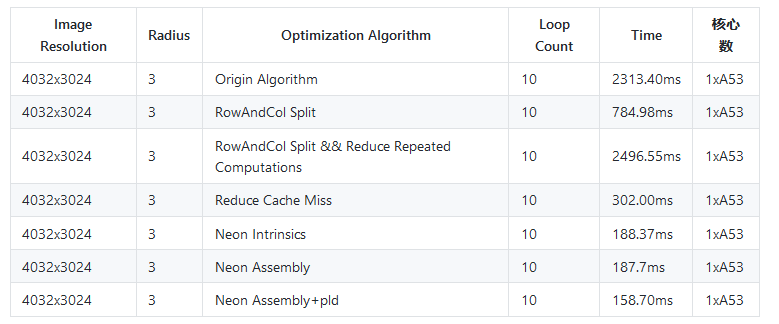可以看到加入pld之后，速度又提升了20ms！说明之前改写的汇编版本直接在内存和寄存器之间读写数据的确过于耗时。

# 9. 第七版优化¶

for(; n > 0; n--){
float32x4_t colsum = vld1q_f32(tmpColSumPtr);
float32x4_t sub = vld1q_f32(tmpsubPtr);
sum = vsubq_f32(sum, sub);

vst1q_f32(tmpColSumPtr, sum);
vst1q_f32(tmpDestPtr, sum);

tmpsubPtr += 4;
tmpColSumPtr += 4;
tmpDestPtr += 4;
}


//处理出所有的差值
asm volatile(
"0:                       \n" //开头0标记，类似do while中的while(n>0)里的0
"pld      [%0, #128]      \n"
"vld1.s32 {d0-d1}, [%0]!  \n"
"pld      [%1, #128]      \n"
"vld1.s32 {d2-d3}, [%1]!  \n"
"vsub.f32 q2, q0, q1      \n"
"vst1.s32 {d4-d5}, [%2]!  \n"
"subs %3, #1              \n"
"bne  0b                  \n"
// OutputOperands
"=r"(tmpsubPtr),
"=r"(tmpDiffPtr),
"=r"(nn)
// InputOperands
"1"(tmpsubPtr),
"2"(tmpDiffPtr),
"3"(nn)
//Clobbers 这里用到了q0,q1,q2这三个向量寄存器
: "cc", "memory", "q0", "q1", "q2"
);

for(;ree > 0; ree--){
tmpDiffPtr++;
tmpsubPtr++;
}

//把差加回去
asm volatile(
"0:                       \n"
"pld      [%0, #128]      \n"
"vld1.s32 {d0-d1}, [%0]!  \n"
"pld      [%1, #128]      \n"
"vld1.s32 {d1-d2}, [%1]   \n"

"vst1.s32 {d4-d5}, [%1]!  \n"
"vst1.s32 {d4-d5}, [%2]!  \n"
"subs %3, #1              \n" //n-=1
"bne  0b                  \n"
// OutputOperands
: "=r"(tmpDiffPtr),
"=r"(tmpColSumPtr),
"=r"(tmpDestPtr),
"=r"(n)
// InputOperands
: "0"(tmpDiffPtr),
"1"(tmpColSumPtr),
"2"(tmpDestPtr),
"3"(n)
//Clobbers 这里用到了q0,q1,q2这三个向量寄存器
: "cc", "memory", "q0", "q1", "q2"
);

for(;re > 0; re--){
*tmpColSumPtr += *tmpDiffPtr;
*tmpDestPtr += *tmpDiffPtr;
tmpDiffPtr++;
tmpDestPtr++;
tmpColSumPtr++;
}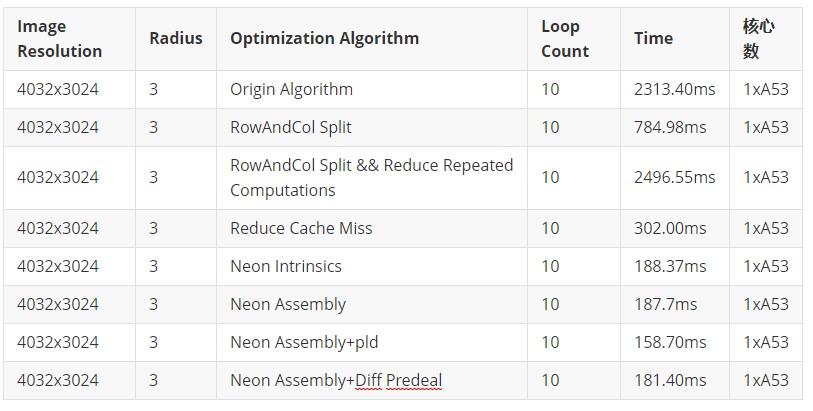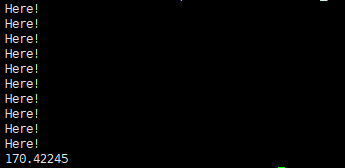# 10. 第八版优化 双发射流水线¶

"pld      [%1, #256]      \n"
"vld1.s32 {d4-d7}, [%1]!  \n" //q2,q3


asm volatile(
"0:                       \n"
"pld      [%0, #256]      \n"
"vld1.s32 {d0-d3}, [%0]!  \n" //q0,q1
"pld      [%2, #256]      \n"
"vld1.s32 {d8-d11}, [%2]  \n" //q4,q5

"pld      [%1, #256]      \n"
"vld1.s32 {d4-d7}, [%1]!  \n" //q2,q3

"vsub.f32 q6, q6, q2      \n"

"vsub.f32 q7, q7, q3      \n"

"vst1.s32 {d12-d15}, [%3]!  \n"//q8, q9

"vst1.s32 {d12-d15}, [%2]!  \n"

"subs %4, #1              \n"
"bne  0b                  \n"

// OutputOperands
"=r"(tmpsubPtr),
"=r"(tmpColSumPtr),
"=r"(tmpDestPtr),
"=r"(n)
// InputOperands
"1"(tmpsubPtr),
"2"(tmpColSumPtr),
"3"(tmpDestPtr),
"4"(n)
//Clobbers 这里用到了q0,q1,q2,q3,q4这五个向量寄存器
: "cc", "memory", "q0", "q1", "q2", "q3", "q4", "q5", "q6", "q7", "q8", "q9"
);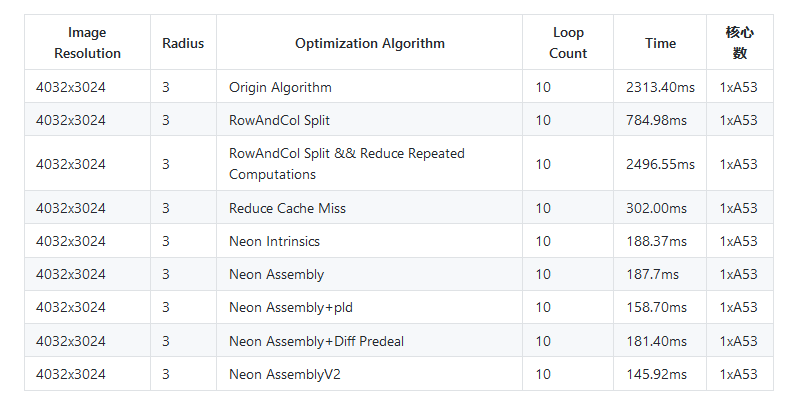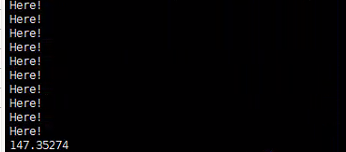# 12. 参考¶